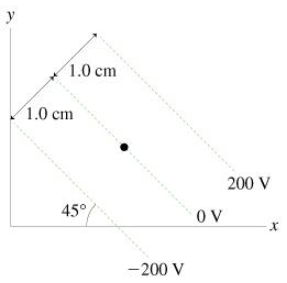# Problem: (A) What is the magnitude of the electric field at the dot in the figure? Express your answer using two significant figures.E = _________V/m(B) What is the direction of the electric field at the dot in the figure? Choose best answer.(a) the negative x-axis.(b) the positive x-axis.(c) 45 below -x-axis(d) 45 below +x-axis

###### FREE Expert Solution

A)

$\overline{){\mathbf{E}}{\mathbf{=}}\frac{\mathbf{V}}{\mathbf{d}}}$

E = 200 V

94% (346 ratings)###### Problem Details(A) What is the magnitude of the electric field at the dot in the figure? Express your answer using two significant figures.
E = _________V/m

(B) What is the direction of the electric field at the dot in the figure? Choose best answer.

(a) the negative x-axis.

(b) the positive x-axis.

(c) 45 below -x-axis

(d) 45 below +x-axis